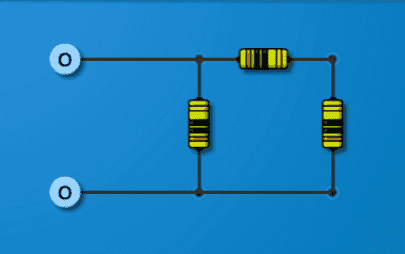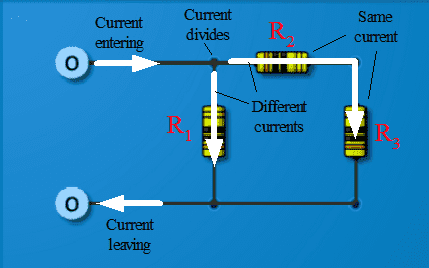# How to find equivalent resistance of this circuit?

## Homework Statement

http://imgur.com/vUoRrhO[Note: Image copy inserted by moderator]

## Homework Equations

Req for parallel is 1/Req=1/R+1/R...

## The Attempt at a Solution

I have never seen a circuit like this and I'm not sure as to how I would go about calculating it since there is a resistor inside of the parallel section. All those resistors are 10 ohms.

Last edited by a moderator:

berkeman
Mentor

## Homework Statement

http://imgur.com/vUoRrhO

## Homework Equations

Req for parallel is 1/Req=1/R+1/R...

## The Attempt at a Solution

I have never seen a circuit like this and I'm not sure as to how I would go about calculating it since there is a resistor inside of the parallel section. All those resistors are 10 ohms.
Combine the two series resistors first (what is the equation for combining series resistors?), and then combine the two resulting parallel resistors.Combine the two series resistors first (what is the equation for combining series resistors?), and then combine the two resulting parallel resistors.Isn't there only one series resistor? I'm still not sure how to do this...

NascentOxygen
Staff Emeritus
Two resistors are in series if all the current that passes through one also passes through the second.

Two resistors are in series if all the current that passes through one also passes through the second.
Ok ok I see now. So the resistor on top is added with the one on the right or left then that is put in parallel with the other one... Still kind of weird though because after adding those 2 resistors, wouldn't it not be in parallel after that?

cnh1995
Homework Helper
Gold Member
So the resistor on top is added with the one on the right or left
Right or left? Which one is in series with the resistor on top?

NascentOxygen
Staff Emeritus
The current enters and leaves this circuit via the terminals on the left.

After adding two resistances the resultant may be in parallel with a third. Perform this simplification in steps, so you don't get confused.

gneill
Mentor
Here's a picture that might help with the discussion:Edit: Added resistor identification labels (red).

Last edited:
Right or left? Which one is in series with the resistor on top?
I think the right.

epenguin
Homework Helper
Gold Member
Was this question meant to test knowledge of resistor colour coding (which I am long unfamiliar with - does it still exist?), the actual calculation being assumed trivial?berkeman
Mentor
I think the right.
Yes. So what do you get for the answer? Please show your work.Yes. So what do you get for the answer? Please show your work.I already have the answer I found it... I'm just confused as to why adding the 2 10 resistors would then put that in parallel with the last one.

Was this question meant to test knowledge of resistor colour coding (which I am long unfamiliar with - does it still exist?), the actual calculation being assumed trivial?No this is just something I made on a gizmo. It's part of a problem I am doing.

cnh1995
Homework Helper
Gold Member
I'm just confused as to why adding the 2 10 resistors would then put that in parallel with the last one.
Adding the two 10 ohm resistors will give series equivalent resistance Req of them. How are Req and the remaining 10 ohm resistor connected?

gneill
Mentor
I already have the answer I found it... I'm just confused as to why adding the 2 10 resistors would then put that in parallel with the last one.
I've added unique identifying labels to the resistors in the figure in post #8. Can you tell us which two resistors you added together and which is the "last one" that you combined with the sum?

Add the series (top and right) use ohms law to get whatever value that you are missing (I'm assuming that you have one of the other values probably the applied voltage). Then use ohms law to solve for the last resistor on the left. This is a series-parrallel circuit and is easiest to solve when broken down into two separate equations.

I've added unique identifying labels to the resistors in the figure in post #8. Can you tell us which two resistors you added together and which is the "last one" that you combined with the sum?
R3 and R2 as series then that with R1 as parallel.

gneill
Mentor
Add the series (top and right) use ohms law to get whatever value that you are missing (I'm assuming that you have one of the other values probably the applied voltage). Then use ohms law to solve for the last resistor on the left. This is a series-parrallel circuit and is easiest to solve when broken down into two separate equations.
I believe that you've misunderstood the problem. It is an entry level problem with three resistors, all 10 Ω. The goal is to reduce the given network to a single equivalent resistance value. It requires the concepts of recognizing series and parallel pairs of resistances and combining said pairs into single values. Eventually a single value representing the entire network's equivalent resistance is achieved.

gneill
Mentor
R3 and R2 as series then that with R1 as parallel.
Correct.

My bad, I wasn't aware that I couldn't provide a complete algebraic solution. My post was deleted, I really need to read the rules. Add the two in series, that leaves you with two resistors that you can use the equation for parallel resistors to find the total resistance. Good luck